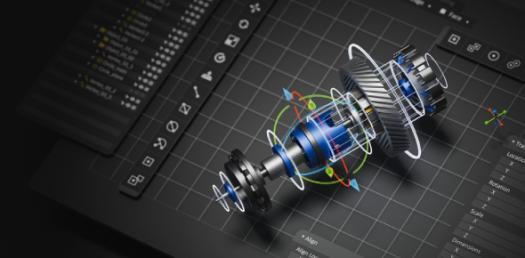# Cads 5850 Lab 1 Quiz Created By Siyuan

10 Questions | Attempts: 316
ShareSettingsPlease take the quiz after you study the excel tutorial sheet.

• 1.
True or false: Excel is like an electronic version of a paper ledger. If you are familiar with Word, a spreadsheet is similar to working with tables—it has rows and columns, and the space where these two intersect are called “cells”.
• A.

True

• B.

False

• 2.
Please check the types of data may occur in excel "cell" (check all possible answers)
• A.

Label

• B.

Mathematical equations

• C.

Text sentence

• D.

Numerical number

• E.

Formula

• F.

None of above

• 3.
Formulas or functions must begin with ____________.
• A.

\$

• B.

*

• C.

=

• D.

@

• E.

!

• F.

#

• 4.
A B C 1 2     2 2 = SUM (A2:A4, A6)   3 3     4 5     5 1     6 3     What is the value of B2?
• A.

9

• B.

10

• C.

12

• D.

15

• E.

13

• 5.
A B 1 2 3 2 3 2 3 1 2 4 2 2 5 1 =SUM (A1:A3) +\$B\$3 Please type in the value of B5 according to the given formula.
• 6.
__________allows  text to span across multiple columns.
• A.

Merge & Center

• B.

Currency

• C.

Wrap Text

• D.

Bold

• E.

Orientation

• 7.
Which formula can add the all the numeric values in a range of cells, ignoring thos​e which are not numeric, and place the result in a different cell?
• A.

Average

• B.

SUM

• C.

MEAN

• D.

COS

• E.

MAX

• 8.
What type of cell reference is this:\$A\$1.
• A.

Mixed

• B.

Relative

• C.

Absolute

• 9.
What  are used to designate each ROW'S location ? (please input one word)
• 10.
A B 1 3 5 2 4 6   If A5 = (A2*B1)/2, then A5= _______? (please input the number)

## Related TopicsBack to top
×

Wait!
Here's an interesting quiz for you.